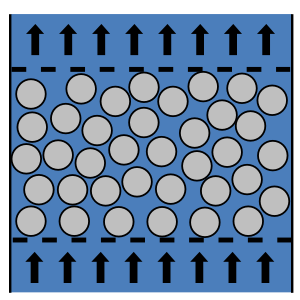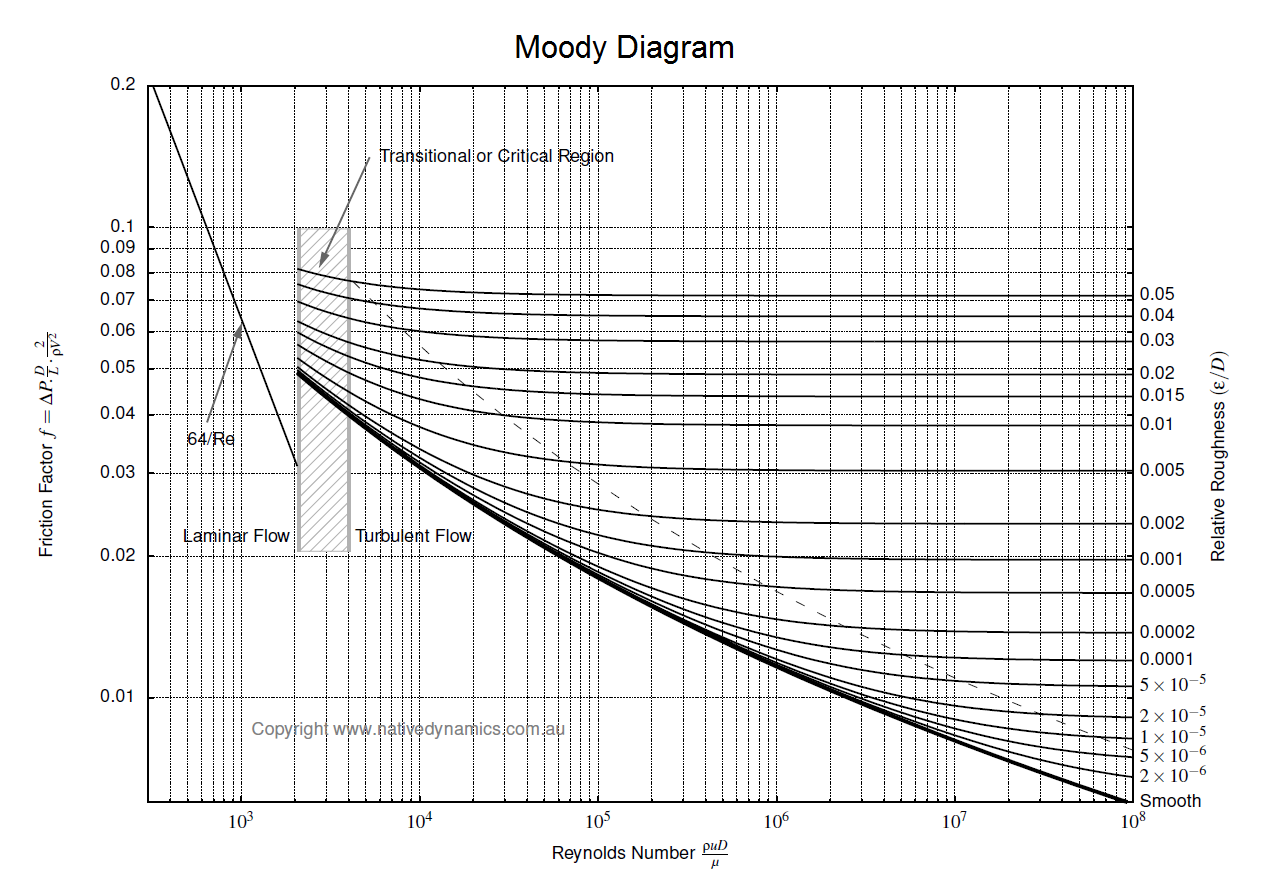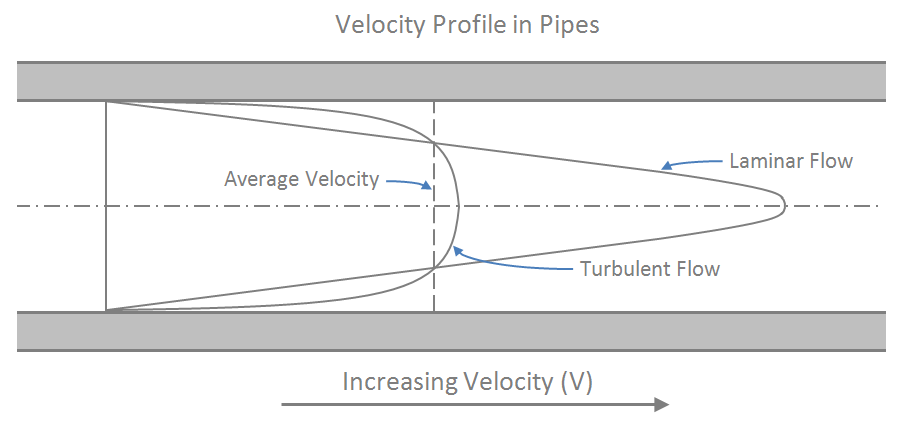f
Neutrium

# Transitional Flow

Packed Bed Reynolds NumberThe packed bed Reynolds number is dimensionless and describes the ratio of inertial to viscous forces for fluid flow through a packed bed. It may be used to calculate the pressure drop though a packed bed via the Ergun equation or identify the boundaries of flow regimes (laminar, transitional and turbulent) in a packed bed. This article will show you how to calculate and interpret the packed bed Reynolds number.

Pressure Loss in PipeTo determine the pressure loss or flow rate through pipe knowledge of the friction between the fluid and the pipe is required. This article describes how to incorporate friction into pressure loss or fluid flow calculations. It also outlines several methods for determining the Darcy friction factor for rough and smooth pipes in both the turbulent and laminar flow regime. Finally this article discusses which correlation for pressure loss in pipe is the most appropriate.

Reynolds NumberThe Reynolds number is dimensionless and describes the ratio of inertial forces to viscous forces in a flowing fluid. It is used in many fluid flow correlations and is used to describe the boundaries of fluid flow regimes (laminar, transitional and turbulent). This article will show you how to calculate and interpret the Reynolds number.